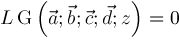﻿ Differential EquationISSAC 97 - Meijer G Function Representations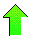Contents News View

 Differential Equation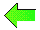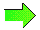Applying products of shift operators to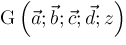we see that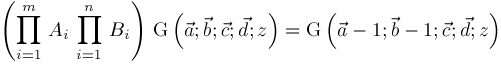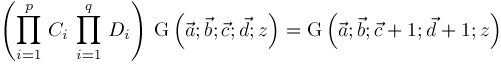It can be checked that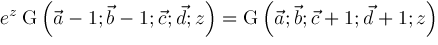Hence,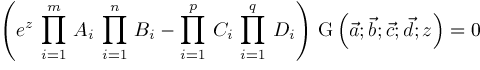Converting to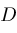notation, we get the differential equation for.  If we let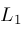,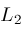, and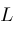be the operators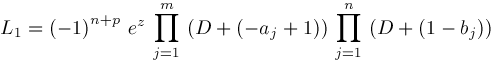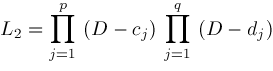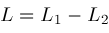then the differential equation forcan be written